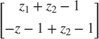# Solve a Linear System of Algebraic Equations

Use the Algebraic Constraint block to solve the systemThe model represents the problem in a vectorized form asThe signal fed to the Algebraic Constraint blockis avector of the formThe block is configured to constrainto 0. Thus solving foryields the solution